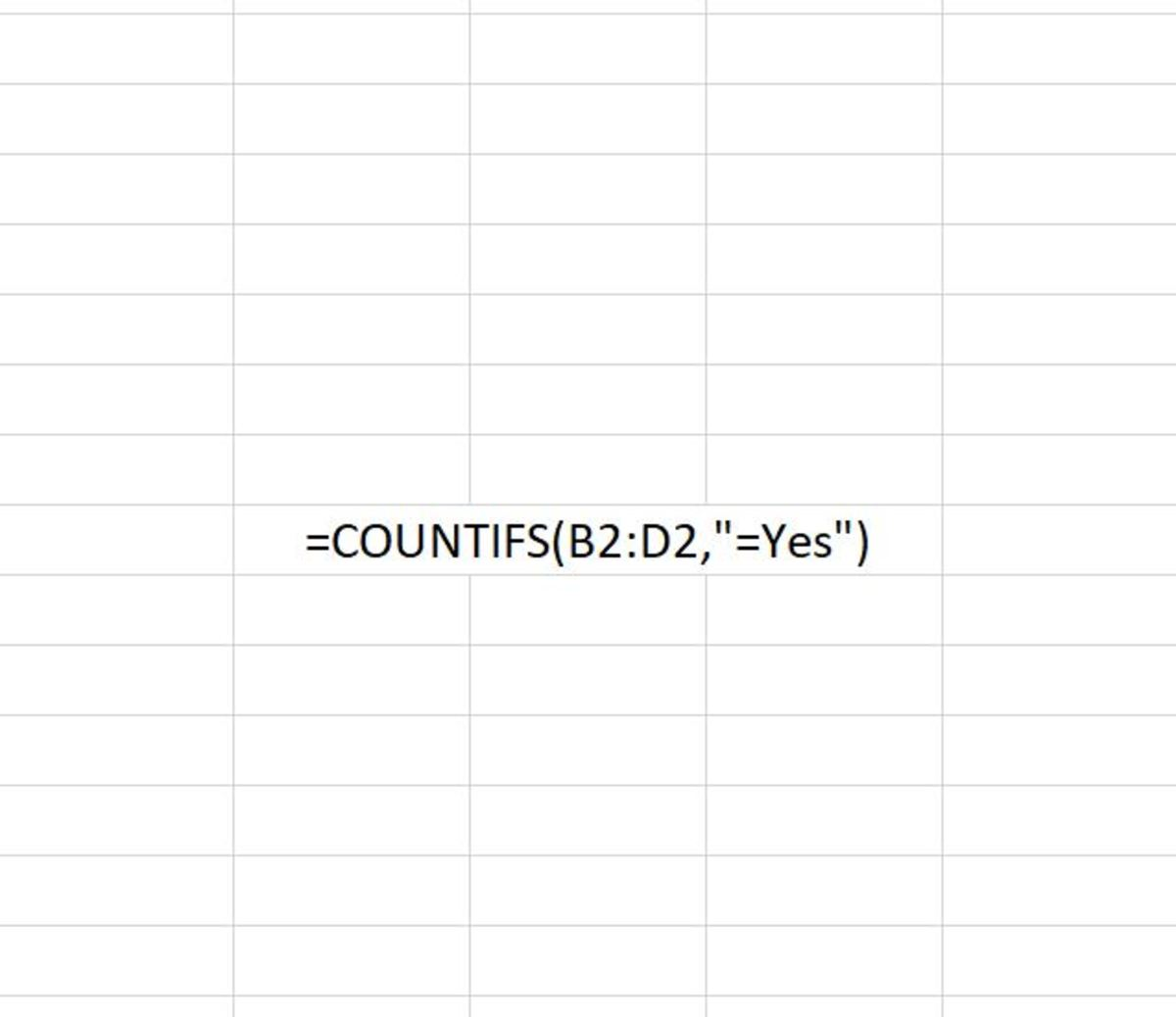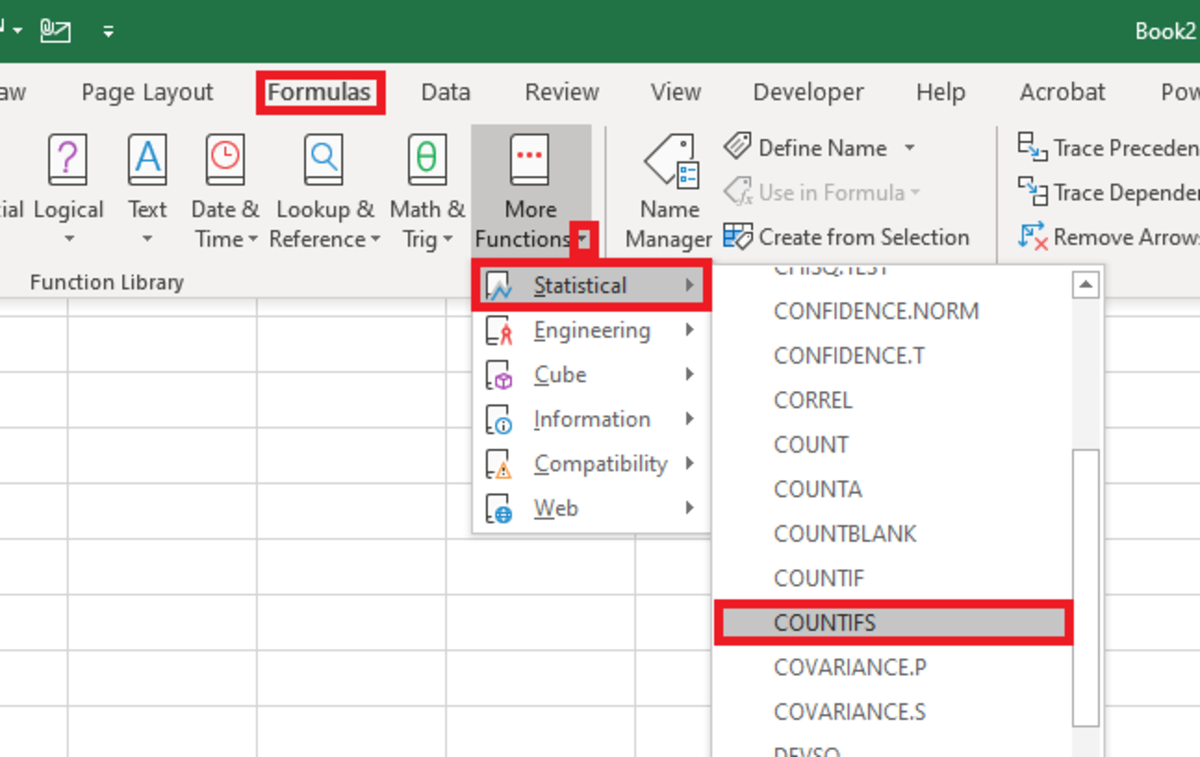# How to Use the COUNTIFS Function in Excel

Joshua earned an MBA from USF and he writes mostly about software and technology.The COUNTIFS function is shown above after typed into an Excel spreadsheet. The formula here is the simplest form of the COUNTIFS function where only one criteria is used to count. Created by Joshua Crowder

## The Purpose of the COUNTIFS Function

The COUNTIFS function counts a number of cells based on a condition or set of conditions. In the latter case, if a set of conditions are met, the function will count it. This function can stand alone or be used within another formula.

There are many applications for this formula so its best for any Excel user to become familiar with its functionality. Here is a sample example of how the COUNTIFS function can be used in a spreadsheet:

• Counting the number of sales regions are of a certain area and are greater than a specific amount.

## COUNTIFS Syntax

The COUNTIFS function needs to be entered in to a cell like a formula. To properly enter this function into a cell, the following steps can be followed:

1. Click on cell.

2. Start the function with "=COUNTIFS(".

3. The first criteria range needs to be added and followed by a comma.

4. The criteria in that range must be added (followed by a comma if more criteria is used).

5. Following, additional criteria ranges and criteria can be added and ended with a closed parenthesis.

The syntax for this function is further detailed below:

=COUNTIFS(criteria_range1, criteria1, [criteria_range2, criteria2]…)

For this function to work, it needs to have at least a criteria and a range. The limit of the amount condition possibilities that can be used is 127. Each criteria will evaluate whether or not the formula can incrementally add another cell to the count or not. The syntax of the function is explained further below:

• Criteria_range1: This argument is a requirement. The first range in which to evaluate the associated criteria.
• Criteria1: This argument is also a requirement. This criteria can be in the form of a number, expression, cell reference, or text that defines which cells will be counted. For example, criteria can be expressed as 32, ">32", B4, "apples", or "32".
• criteria_range2, criteria2: Additional criteria can be added but is totally optional and followed by their respective criteria.

The COUNTIFS function can be inserted into cell with the use of an insert tool for functions. To use this method:

Scroll to Continue
• Select a cell where you need to function to appear.
• Next, navigate to the formulas tab and click on the "More Functions" button.
• Choose the statistical selection from the list is then chosen and find COUNTIFS from the list of functions.

## Selecting the COUNTIFS FunctionTo insert the COUNTIFS function from the formula tab you must first navigate to: Formulas→More Functions→Statistical→COUNTIFSCreated by Joshua Crowder
• After the the functions arguments window appears, criteria ranges and criteria can be added. Once a range and criteria are added, additional fields will be added.
• Each time the conditions are met the function will add to the count in the result.

## COUNTIFS Function Examples

Here are some examples of how the COUNTIFS function can be used:

=COUNTIFS(B2:B7,">0")

One Criteria: Here the minimum of one criteria is used to count. In the range reference B2:B7, for each cell that has a value greater than zero, each will be tallied.

=COUNTIFS(range1,">0")

One Criteria: Here the minimum of one criteria is used to count in a named range.. In the named range cells will be counted that have a value greater than zero.

=COUNTIFS(B2:B7,">0", C2:C7,"=0")

Multiple Criteria: Here multiple criteria are used to count data in multiple ranges. In the range reference B2:B7 cells that have a value greater than zero and cells in range C2:C7 will be counted if the values equal zero.

## COUNTIFS Function Practical Example

Here I cover a simple example on how the COUNTIFS function with two criteria. The insert function option will be used to insert the function arguments.

The data below show one column with zeros and the next column with letters. I would like to count the instances where a zero and J occur side-by-side.

Using the function insertion tool, I add each range with the requirement to be counted be that they equal zero or J.

The results shows that there are three instances as I have highlighted below.

To learn more about using functions in Excel I recommend The Excel 2019 Bible book. I have been using the Excel Bible for years to improve my understanding of all aspects of this Microsoft product.

Microsoft. (n.d.). COUNTIFS function. Retrieved January 5, 2020, from https://support.office.com/en-us/article/countifs-function-dda3dc6e-f74e-4aee-88bc-aa8c2a866842.

How to Use the COUNT Function in Excel

How to Use the AVERAGE Function in Excel

How to Use the ABS Function in Excel

How to Remove Errors in Excel With the IFERROR Function

How to Use the VLOOKUP Function in Excel

Converting Measurement Units: Using the CONVERT Function

This content is accurate and true to the best of the author’s knowledge and is not meant to substitute for formal and individualized advice from a qualified professional.